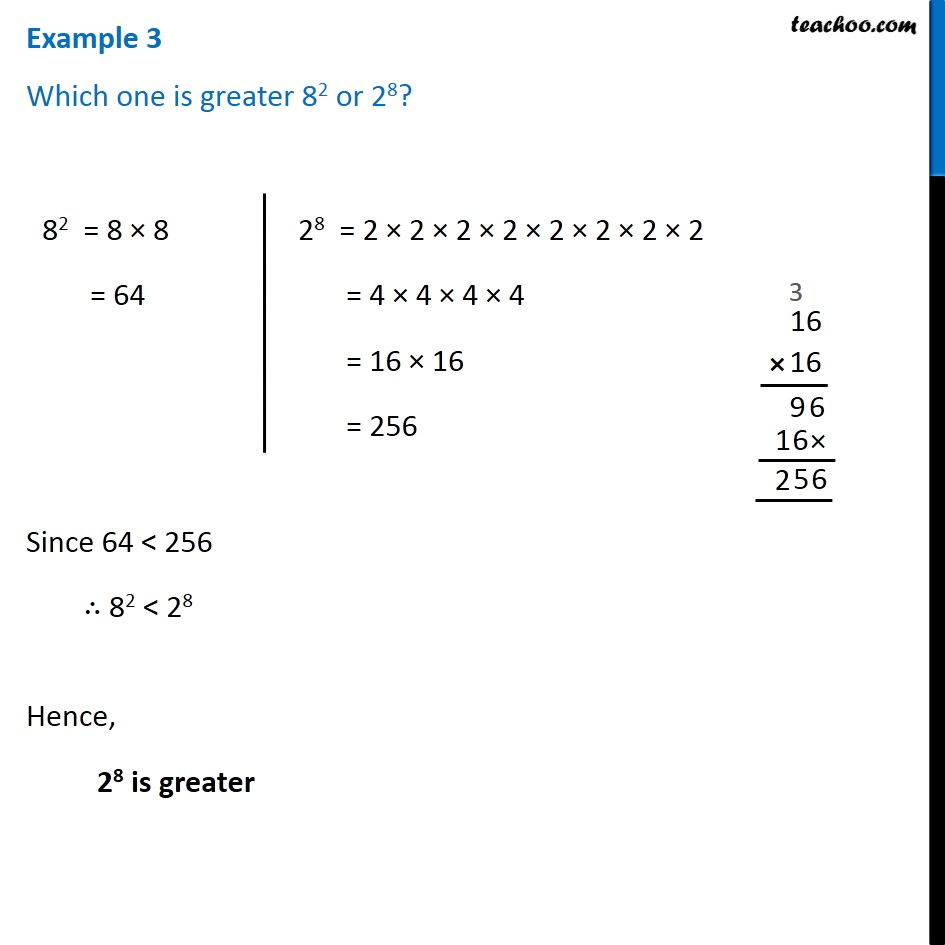Subscribe to our Youtube Channel - https://you.tube/teachoo

1. Chapter 13 Class 7 Exponents and Powers
2. Serial order wise
3. Examples

Transcript

Example 3 Which one is greater 82 or 28? 82 = 8 × 8 = 64 28 = 2 × 2 × 2 × 2 × 2 × 2 × 2 × 2 = 4 × 4 × 4 × 4 = 16 × 16 = 256 Since 64 < 256 ∴ 82 < 28 Hence, 28 is greater

Examples